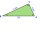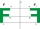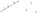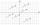# Line + vector - math problems

#### Number of problems found: 18

• Space vectors 3DThe vectors u = (1; 3; -4), v = (0; 1; 1) are given. Find the size of these vectors, calculate the angle of the vectors, the distance between the vectors.
• Perpendicular projectionDetermine the distance of a point B[1, -3] from the perpendicular projection of a point A[3, -2] on a straight line 2 x + y + 1 = 0.
• Place vectorPlace the vector AB, if A (3, -1), B (5,3) in the point C (1,3) so that AB = CO
• Ascend vs. descentWhich function is growing? a) y = 2-x b) y = 20 c) y = (x + 2). (-5) d) y = x-2
• Three pointsThree points K (-3; 2), L (-1; 4), M (3, -4) are given. Find out: (a) whether the triangle KLM is right b) calculate the length of the line to the k side c) write the coordinates of the vector LM d) write the directional form of the KM side e) write the d
• Calculate 6Calculate the distance of a point A[0, 2] from a line passing through points B[9, 5] and C[1, -1].
• DodecagonCalculate the size of the smaller of the angles determined by lines A1 A4 and A2 A10 in the regular dodecagon A1A2A3. .. A12. Express the result in degrees.
• Decide 2Decide whether points A[-2, -5], B[4, 3] and C[16, -1] lie on the same line
• Coordinates of a centroindLet’s A = [3, 2, 0], B = [1, -2, 4] and C = [1, 1, 1] be 3 points in space. Calculate the coordinates of the centroid of △ABC (the intersection of the medians).
• Parametric formCalculate the distance of point A [2,1] from the line p: X = -1 + 3 t Y = 5-4 t Line p has a parametric form of the line equation. ..
• Find the 10Find the value of t if 2tx+5y-6=0 and 5x-4y+8=0 are perpendicular, parallel, what angle does each of the lines make with the x-axis, find the angle between the lines?
• Find theFind the image A´ of point A [1,2] in axial symmetry with the axis p: x = -1 + 3t, y = -2 + t (t = are real number)
• Find the 5Find the equation of the circle with center at (1,20), which touches the line 8x+5y-19=0
• Two peopleTwo straight lines cross at right angles. Two people start simultaneously at the point of intersection. John walking at the rate of 4 kph in one road, Jenelyn walking at the rate of 8 kph on the other road. How long will it take for them to be 20√5 km apa
• Points collinearShow that the point A(-1,3), B(3,2), C(11,0) are col-linear.
• Three points 2The three points A(3, 8), B(6, 2) and C(10, 2). The point D is such that the line DA is perpendicular to AB, and DC is parallel to AB. Calculate the coordinates of D.
• Parametric equationsWrite the parametric equations of height hc in triangle ABC: A = [5; 6], B = [- 2; 4], C = [6; -1]
• LineLine p passing through A[-10, 6] and has direction vector v=(3, 2). Is point B[7, 30] on the line p?

Do you have an exciting math question or word problem that you can't solve? Ask a question or post a math problem, and we can try to solve it.

We will send a solution to your e-mail address. Solved examples are also published here. Please enter the e-mail correctly and check whether you don't have a full mailbox.

Our vector sum calculator can add two vectors given by their magnitudes and by included angle. Line - math problems. Vector - math problems.